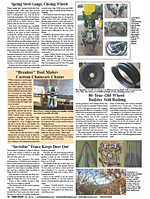Use Google Earth To Measure The Acreage of Fields
I've found a way to use Google Earth, which is a free program you can download at www.earth.google.com, to measure the acreage of fields.
After finding the field, you just click on the "measure" logo on the toolbar. Change "mile" to "feet". If the field has rounded edges, then change to path. Start going around the field with the cursor. Keep the line following the edge of the field. Go completely around the boundary. Take the figure and divide it by 4. Then take that number and multiply it by that same number. Then divide that number by 43,560.
For example, if the total number is 4,000 ft., divide that by 4 and the answer is 1,000. Then multiply 1,000 by 1,000 which equals 1,000,000. Divide that by 43,560, which equals 22.9 acres. It's very accurate. We were extending a terrace and measured first on the computer. When we got to the field we were only off by 2 ft. (Glen Schweppe, Syracuse, Neb. 68446)